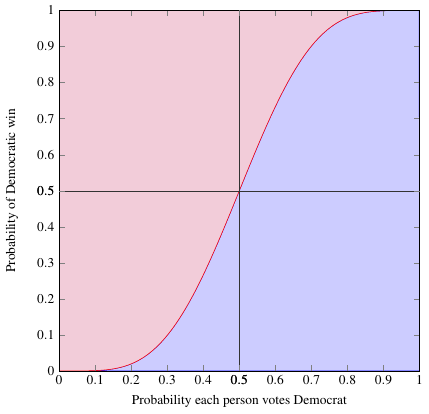1. 2012
Nov
06

## The win-more effect of indirect electionsIt’s Election Day (in the US), and I have a relevant post I’ve been meaning to do for a while.

Suppose you have a binary experiment, one which has two possible outcomes with probabilities $$p$$ and $$q = 1-p$$. For example, voting. (Pretend there are only 2 parties) Overall, let’s say people vote Democrat with probability $$p$$ and Republican with probability $$q$$. Now suppose a large number $$N$$ of people all go out to vote; what can you say about the results?

In a statistical experiment like this, the possible results are drawn from a binomial distribution, in which the probability of getting $$n$$ Democratic votes (and $$N - n$$ Republican) is

$$P(n) = \binom{N}{n}p^n q^{N-n}$$

The probability that the Democrats will come out ahead is just the sum of all the probabilities for all the outcomes where $$n$$ is more than half of the total vote: we start at $$n = \floor*{\frac{N}{2} + 1}$$, which is the first integer greater than $$\frac{N}{2}$$, and add up probabilities all the way to $$n = N$$.

P_D(N,p) = \sum_{n=\floor*{N/2 + 1}}^{N}\binom{N}{n}p^n q^{N-n} = 1 …